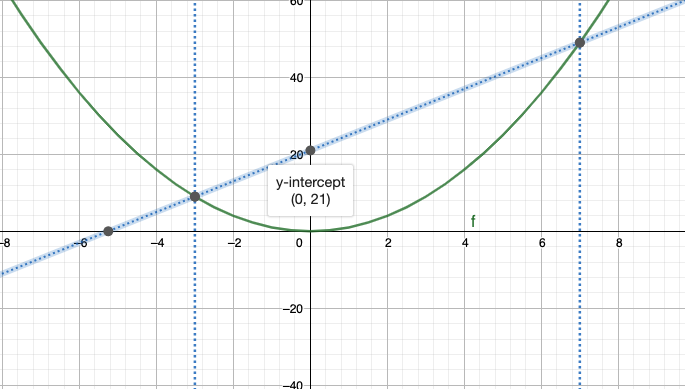### how to use a parabola for multiplication maths

It's possible to multiply any two real numbers using just a parabola and some straight lines. Pick two numbers, x1 and x2 say, extend a line vertically until you hit the parabola, join both of these points and where this line intersects the y-axis, is the product -ax1x2, where a is the coefficient of the x2 term in the parabola.

In the example below, I choose x1 = 7 and x2 = -3, and a parabola described by y = x2.
As you can see, the line joining points (-3,9) and (7,49) intersects the y-axis at (0,21), which is equal to -(1)(7)(3).The choice of parabola is irrelevant here, as long as the constant term is 0. I will use the general from of a parabola with no constant term, that is y = ax2+bx, in the proof below to demonstrate this.

Proof:
Claim: The y-intersect of the line joining two points on a parabola described by y = ax2+bx is the negative of the product of the x components of the same two points on the parabola and the coefficient of the x2 term describing the parabola.

Let x1 and x2 be two arbitrary points on the x-axis. Then, both

y1 = ax12+bx1
y2 = ax22+bx2

are points lying on the parabola, and the equation of the line joining them is given by l = mx+c, where m is the slope and is described by:

m = (1/x1-x2)(ax12+bx1 - (ax22+bx2))
m = (1/x1-x2)(ax12+bx1 - ax22-bx2)
m = (1/x1-x2)(a(x12-x22)+b(x1-x2))
m = (1/x1-x2)(a(x1-x2)(x1+x2)+b(x1-x2)) (Difference of squares)
m = a(x1+x2)+b

Therefore, the line l joining y1 and y2 on the parabola is given by l = (a(x1+x2)+b)x+c. It just remains to calculate the value of c, which is the y-intersect of l.

Since we already know that (x1,ax12+bx1) is a point on the parabola, filling these values into the equation of l, we get:

ax12+bx1 = (a(x1+x2)+b)x1+c
ax12+bx1 = ax12+ax1x2+bx1+c
0 = ax1x2+c
c = -ax1x2

Note:
The parabola can't have a non-zero constant term, as this value would just shift the parabola up or down vertically, which would only affect the value of the y interesection. For more on parabolas, Matt Parker has a video on his youtube channel: There is only One True Parabola